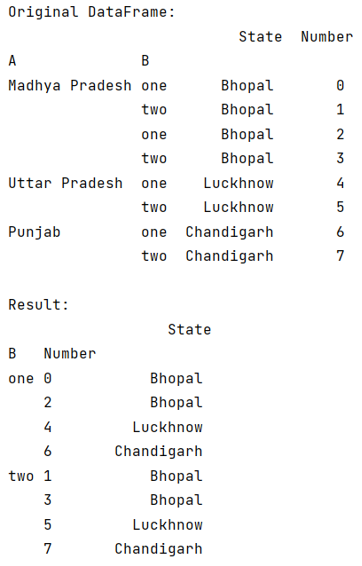# Python - Group by index and column in pandas

Given a Pandas DataFrame, we have to group by index and column in pandas.
Submitted by Pranit Sharma, on August 05, 2022

Pandas is a special tool that allows us to perform complex manipulations of data effectively and efficiently. Inside pandas, we mostly deal with a dataset in the form of DataFrame. DataFrames are 2-dimensional data structures in pandas. DataFrames consist of rows, columns, and data.

Index in pandas is just the number of rows defined in a Series or DataFrame. The index always starts from 0 to n-1 where n is the number of rows. The index is used in indexing which is a method of traversing or selecting particular rows and columns or selecting all the rows and columns.

Columns are the different fields that contain their particular values when we create a DataFrame. We can perform certain operations on both rows & column values.

To group by index and columns, we will first create a multiindex dataframe, then we will groupby index and columns.

Let us understand with the help of an example,

## Python code to group by index and column in pandas

```# Importing pandas package
import pandas as pd

# Importing numpy package
import numpy as np

# Creating arrays for making index

['one', 'two', 'one', 'two', 'one', 'two', 'one', 'two']]

index = pd.MultiIndex.from_arrays(arrays, names=['A', 'B'])

# Creating a DataFrame
df = pd.DataFrame({'State': ['Bhopal','Bhopal','Bhopal','Bhopal','Luckhnow','Luckhnow','Chandigarh','Chandigarh'],
'Number': np.arange(8)}, index=index)

# Display original DataFrame
print("Original DataFrame:\n",df,"\n")

# Groupby function
result = df.groupby(['B', 'Number']).sum()

# Print result
print("Result:\n",result)
```

Output:Languages: » C » C++ » C++ STL » Java » Data Structure » C#.Net » Android » Kotlin » SQL
Web Technologies: » PHP » Python » JavaScript » CSS » Ajax » Node.js » Web programming/HTML
Solved programs: » C » C++ » DS » Java » C#
Aptitude que. & ans.: » C » C++ » Java » DBMS
Interview que. & ans.: » C » Embedded C » Java » SEO » HR
CS Subjects: » CS Basics » O.S. » Networks » DBMS » Embedded Systems » Cloud Computing
» Machine learning » CS Organizations » Linux » DOS
More: » Articles » Puzzles » News/Updates